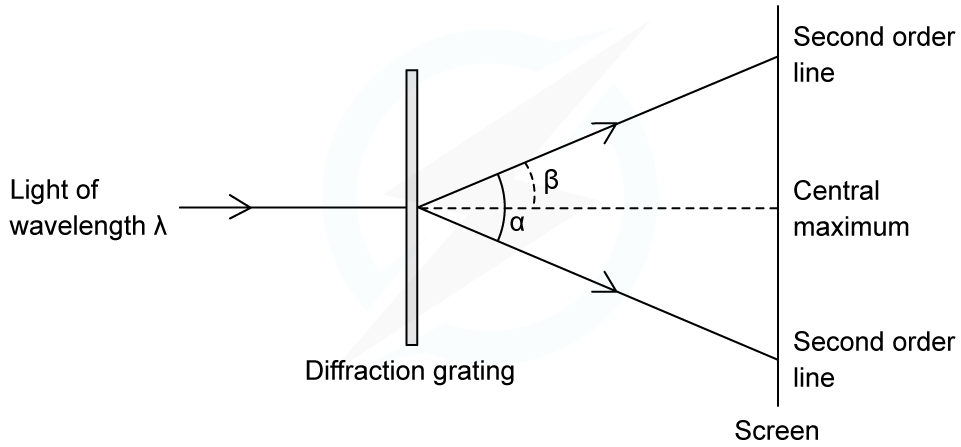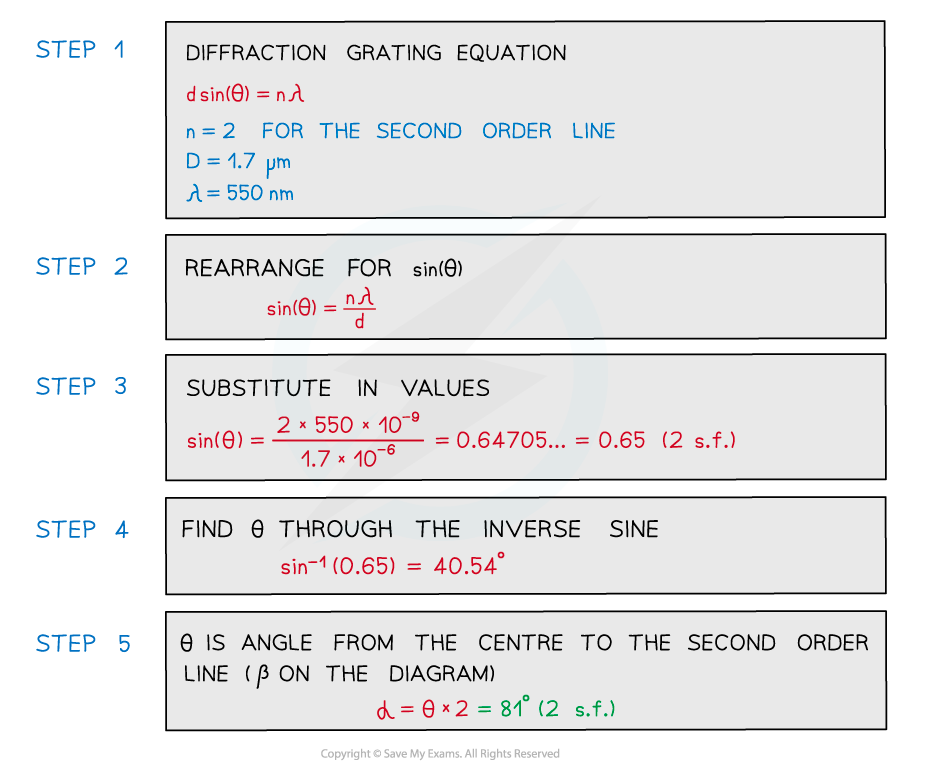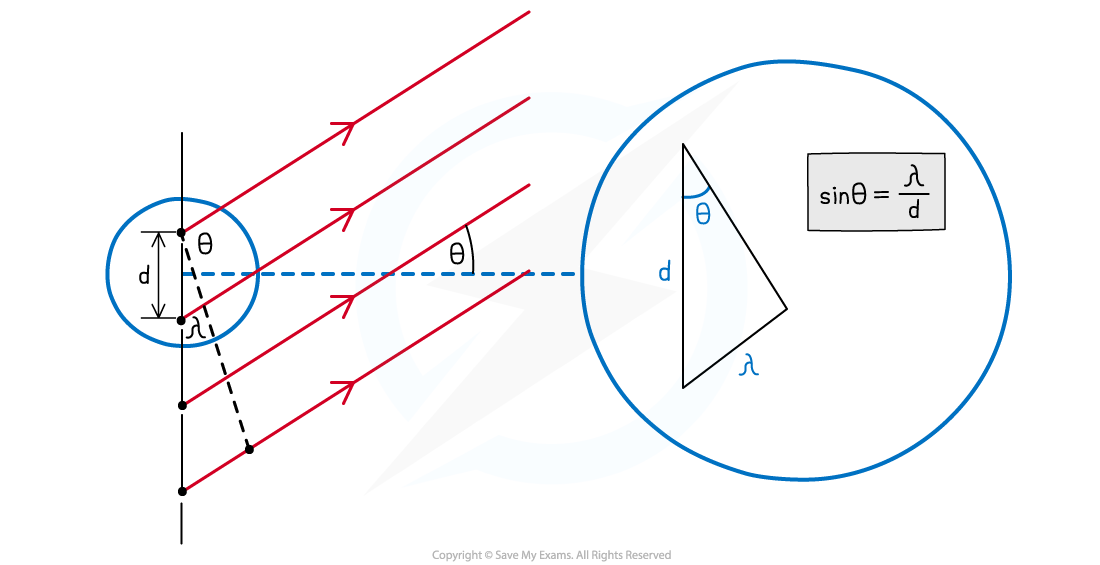# IB DP Physics: HL复习笔记9.3.4 The Diffraction Grating Equation

### The Diffraction Grating Equation

• The angles at which the maxima of intensity (constructive interference) are produced can be deduced by the diffraction grating equation:• The lines per m (or per mm, per nm etc.) on the grating is usually represented by the symbol N
• Therefore, the spacing between each slit, d, can be calculated from N using the equation:#### Angular Separation

• The angular separation of each maxima is calculated by rearranging the grating equation to make θ the subject
• The angle θ is taken from the centre meaning the higher orders are at greater anglesAngular separation

• The angular separation between two angles is found by subtracting the smaller angle from the larger one
• The angular separation between the first and second maxima n1 and nis θ2 – θ1

#### Orders of Maxima

• The maximum angle to see orders of maxima is when the beam is at right angles to the diffraction grating
• This means θ = 90o and sin θ = 1
• The highest order of maxima visible is therefore calculated by the equation:• Note that since n must be an integer, if the value is a decimal it must be rounded down
• E.g If n is calculated as 2.7 then n = 2 is the highest order visible

#### Worked Example

An experiment was set up to investigate light passing through a diffraction grating with a slit spacing of 1.7 µm. The fringe pattern was observed on a screen. The wavelength of the light is 550 nm.Calculate the angle α between the two second-order lines.#### Derivation of the Diffraction Grating Equation

• When light passes through the slits of the diffraction grating, the path difference at the zeroth order maximum is zero
• At the first-order maxima (n = 1), there is constructive interference, hence the path difference is λ
• Therefore, at the nth order maxima, the path difference is equal to nλUsing this diagram and trigonometry, the diffraction grating equation can be derived

• Using trigonometry, an expression for the first order maxima can be written:• Where:
• θ = the angle between the normal and the maxima
• λ = the wavelength of the light (m)
• d = the slit separation (m)
• This means, for n = 1:• Similarly, for n = 2, where the path difference is 2λ:• Therefore, in general, where the path difference is nλ:• A small rearrangement leads to the equation for the diffraction grating:

d sin θn =

#### Exam Tip

Take care that the angle θ is the correct angle taken from the centre and not the angle taken between two orders of maxima.